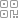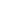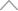• 找新知
• 找服务/产品
• 找课程
• 找LIVE
• 找活动
• 找达人海量新知2 2 4 2 8 8 7

# 以FIFA球员数据集为例，详解3大酷炫可视化技巧· 图形分类相关性

· 散点图矩阵

· 使用Seaborn的分类散点图注释和图注释

import numpy as np

import pandas as pd

import seaborn as sns

import matplotlib.pyplot as plt

%matplotlib inline

# We dont Probably need the Gridlines. Do we? If yes comment this line

sns.set(style="ticks")

numcols = [

'Overall',

'Potential',

'Crossing','Finishing',  'ShortPassing',  'Dribbling','LongPassing', 'BallControl', 'Acceleration',

'SprintSpeed', 'Agility',  'Stamina',

'Value','Wage']

catcols = ['Name','Club','Nationality','Preferred Foot','Position','Body Type']

# Subset the columns

player_df = player_df[numcols+ catcols]

# Few rows of datadef wage_split(x):

try:

return int(x.split("K")[1:])

except:

return 0

player_df['Wage'] = player_df['Wage'].apply(lambda x : wage_split(x))

def value_split(x):

try:

if 'M' in x:

return float(x.split("M")[1:])

elif 'K' in x:

return float(x.split("K")[1:])/1000

except:

return 0

player_df['Value'] = player_df['Value'].apply(lambda x : value_split(x))corr = player_df.corr()

g = sns.heatmap(corr,  vmax=.3, center=0,

square=True, linewidths=.5, cbar_kws={"shrink": .5}, annot=True, fmt='.2f', cmap='coolwarm')

sns.despine()

g.figure.set_size_inches(14,10)

plt.show()1. 数值变量

2. 分类变量3. 数值变量和分类变量

player_df = player_df.fillna(0)

results = associations(player_df,nominal_columns=catcols,return_results=True)· 球员的位置与运球能力高度相关。总不能让梅西踢后卫吧！

· 值与传球和控球的相关性比运球更高。规则是永远传球，正如内马尔的传球。

· “俱乐部”和“收入”有很高的相关性并且可预测。

· “体型”与“踢球偏好的脚”高度相关。这是否意味着如果某球员是瘦子，就很可能喜欢用左脚踢球？这可能没啥实际意义，需要进一步调查。“Anscombe四重奏”由四个相关性几乎近似于1的数据集组成，但具有非常不同的数据分布，并且在绘制时呈现出非常不同的效果。Anscombe四重奏：相关性变化无常

filtered_player_df = player_df[(player_df['Club'].isin(['FC Barcelona', 'Paris Saint-Germain',

'Manchester United', 'Manchester City', 'Chelsea', 'Real Madrid','FC Porto','FC Bayern München'])) &

(player_df['Nationality'].isin(['England', 'Brazil', 'Argentina',

'Brazil', 'Italy','Spain','Germany']))

]

# Single line to create pairplot

g = sns.pairplot(filtered_player_df[['Value','SprintSpeed','Potential','Wage']])· 工资和价值高度相关。

· 大多数其它值也是相关的，然而“潜力”与“价值”的比的趋势是不寻常的。可以看到，当到达特定的潜在阀值时，价值如何呈指数增长。这些信息对建模很有帮助，可以对“潜力”进行转换使其更具有相关性吗？

g = sns.pairplot(filtered_player_df[['Value','SprintSpeed','Potential','Wage','Club']],hue = 'Club')· 波尔图队的工资分配趋向于工资少的那一端。

· 该图无法看出波尔图队球员价值的急剧分布，波尔图队的球员们总是在寻求机会。

· 许多粉红点（代表切尔西队）在“潜力”和“工资”图上形成了一个集群。切尔西队有很多工资较低的高潜力球员，需要更多关注。g = sns.swarmplot(y = "Club",

x = 'Wage',

data = filtered_player_df,

# Decrease the size of the points to avoid crowding

size = 7)

# remove the top and right line in graph

sns.despine()

g.figure.set_size_inches(14,10)

plt.show()g = sns.boxplot(y = "Club",

x = 'Wage',

data = filtered_player_df, whis=np.inf)

g = sns.swarmplot(y = "Club",

x = 'Wage',

data = filtered_player_df,

# Decrease the size of the points to avoid crowding

size = 7,color = 'black')

# remove the top and right line in graph

sns.despine()

g.figure.set_size_inches(12,8)

plt.show()max_wage = filtered_player_df.Wage.max()

max_wage_player = filtered_player_df[(player_df['Wage'] == max_wage)]['Name'].values

g = sns.boxplot(y = "Club",

x = 'Wage',

data = filtered_player_df, whis=np.inf)

g = sns.swarmplot(y = "Club",

x = 'Wage',

data = filtered_player_df,

# Decrease the size of the points to avoid crowding

size = 7,color='black')

# remove the top and right line in graph

sns.despine()

# Annotate. xy for coordinate. max_wage is x and 0 is y. In this plot y ranges from 0 to 7 for each level

# xytext for coordinates of where I want to put my text

plt.annotate(s = max_wage_player,

xy = (max_wage,0),

xytext = (500,1),

# Shrink the arrow to avoid occlusion

arrowprops = {'facecolor':'gray', 'width': 3, 'shrink': 0.03},

backgroundcolor = 'white')

g.figure.set_size_inches(12,8)

plt.show()· 看看该图下方的波尔图队，工资预算如此之小，难以和其它高收入的球队们竞争。

· 皇马和巴塞罗那有许多高薪球员。

· 曼联的工资中位数最高。

· 曼联和切尔西注重平等，许多球员的工资水平都差不多。

· 虽然内马尔比梅西更受重视，但梅西和内马尔的工资差距巨大。

https://towardsdatascience.com/3-awesome-visualization-techniques-for-every-dataset-9737eecacbe8## 新知精选

### 新知榜

1 直播带货2 免税店3 MCN4 特斯拉5 B站6 牛市7 中概股8 ToB9 瑞幸10 新基建•扫码下载商业新知APP~

•回到顶部